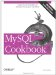# Breaking Down Time Intervals into Components

5.16.1 Problem

You have a time interval represented as a time, but you want the interval in terms of its components.

5.16.2 Solution

Decompose the interval with the HOUR( ), MINUTE( ), and SECOND( ) functions. If the calculation is complex in SQL and you're using the interval within a program, it may be easier to use your programming language to perform the equivalent math.

5.16.3 Discussion

To express a time interval in terms of its constituent hours, minutes, and seconds values, calculate time interval subparts in SQL using the HOUR( ), MINUTE( ), and SECOND( ) functions. (Don't forget that if your intervals may be negative, you need to take that into account.) For example, to determine the components of the intervals between the t1 and t2 columns in the time_val table, the following SQL statement does the trick:

```mysql> SELECT t1, t2,
-> SEC_TO_TIME(TIME_TO_SEC(t2) - TIME_TO_SEC(t1)) AS 'interval as TIME',
-> IF(SEC_TO_TIME(TIME_TO_SEC(t2) >= TIME_TO_SEC(t1)),'+','-') AS sign,
-> HOUR(SEC_TO_TIME(TIME_TO_SEC(t2) - TIME_TO_SEC(t1))) AS hour,
-> MINUTE(SEC_TO_TIME(TIME_TO_SEC(t2) - TIME_TO_SEC(t1))) AS minute,
-> SECOND(SEC_TO_TIME(TIME_TO_SEC(t2) - TIME_TO_SEC(t1))) AS second
-> FROM time_val;
+----------+----------+------------------+------+------+--------+--------+
| t1 | t2 | interval as TIME | sign | hour | minute | second |
+----------+----------+------------------+------+------+--------+--------+
| 15:00:00 | 15:00:00 | 00:00:00 | + | 0 | 0 | 0 |
| 05:01:30 | 02:30:20 | -02:31:10 | - | 2 | 31 | 10 |
| 12:30:20 | 17:30:45 | 05:00:25 | + | 5 | 0 | 25 |
+----------+----------+------------------+------+------+--------+--------+```

But that's fairly messy, and attempting to do the same thing using division and modulo operations is even messier. If you happen to be issuing an interval-calculation query from within a program, it's possible to avoid most of the clutter. Use SQL to compute just the intervals in seconds, then use your API language to break down each interval into its components. The formulas should account for negative values and produce integer values for each component. Here's an example function time_components( ) written in Python that takes an interval value in seconds and returns a four-element tuple containing the sign of the value, followed by the hour, minute, and second parts:

```def time_components (time_in_secs):
if time_in_secs < 0:
sign = "-"
time_in_secs = -time_in_secs
else:
sign = ""
hours = int (time_in_secs / 3600)
minutes = int ((time_in_secs / 60)) % 60
seconds = time_in_secs % 60
return (sign, hours, minutes, seconds)```

You might use time_components( ) within a program like this:

```query = "SELECT t1, t2, TIME_TO_SEC(t2) - TIME_TO_SEC(t1) FROM time_val"
cursor = conn.cursor ( )
cursor.execute (query)
for (t1, t2, interval) in cursor.fetchall ( ):
(sign, hours, minutes, seconds) = time_components (interval)
print "t1 = %s, t2 = %s, interval = %s%d h, %d m, %d s"
% (t1, t2, sign, hours, minutes, seconds)
cursor.close ( )```

The program produces the following output:

```t1 = 15:00:00, t2 = 15:00:00, interval = 0 h, 0 m, 0 s
t1 = 05:01:30, t2 = 02:30:20, interval = -2 h, 31 m, 10 s
t1 = 12:30:20, t2 = 17:30:45, interval = 5 h, 0 m, 25 s```

The preceding example illustrates a more general principle that's often useful when issuing queries from a program: it may be easier to deal with a calculation that is complex to express in SQL by using a simpler query and postprocessing the results using your API language.MySQL Cookbook
ISBN: 059652708X
EAN: 2147483647
Year: 2005
Pages: 412
Authors: Paul DuBois